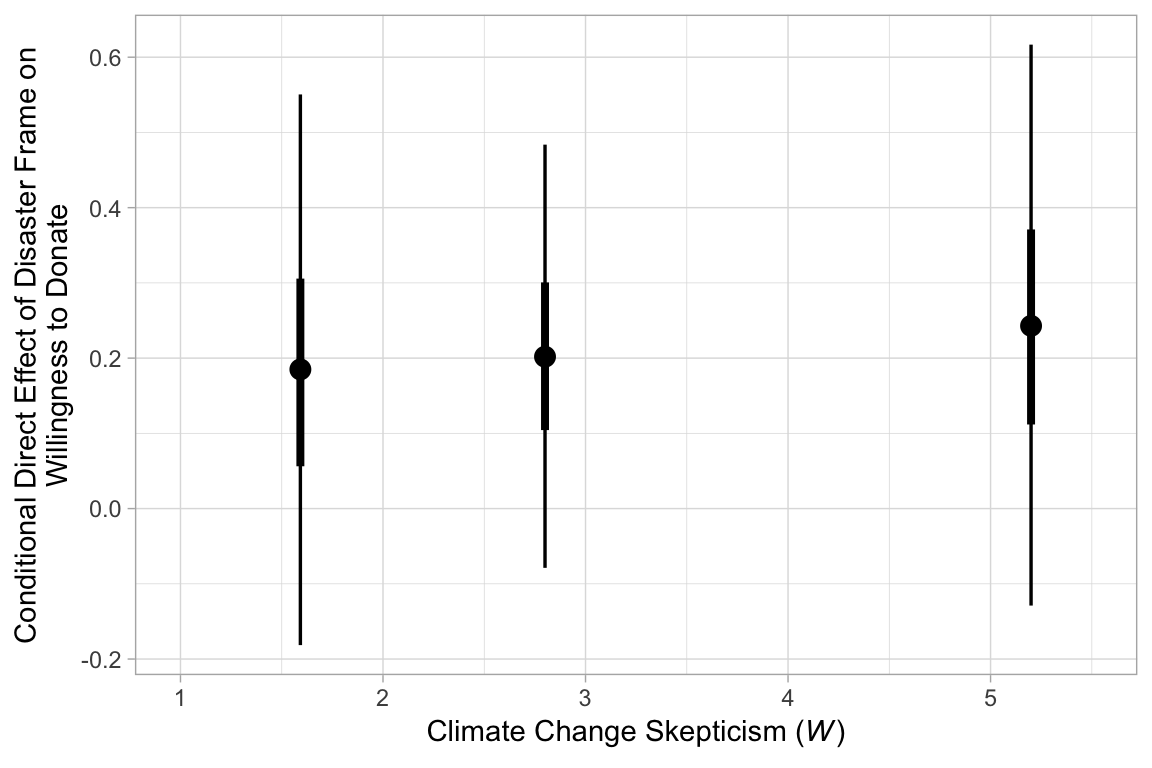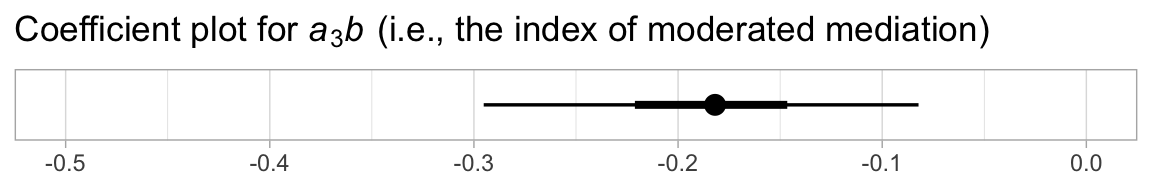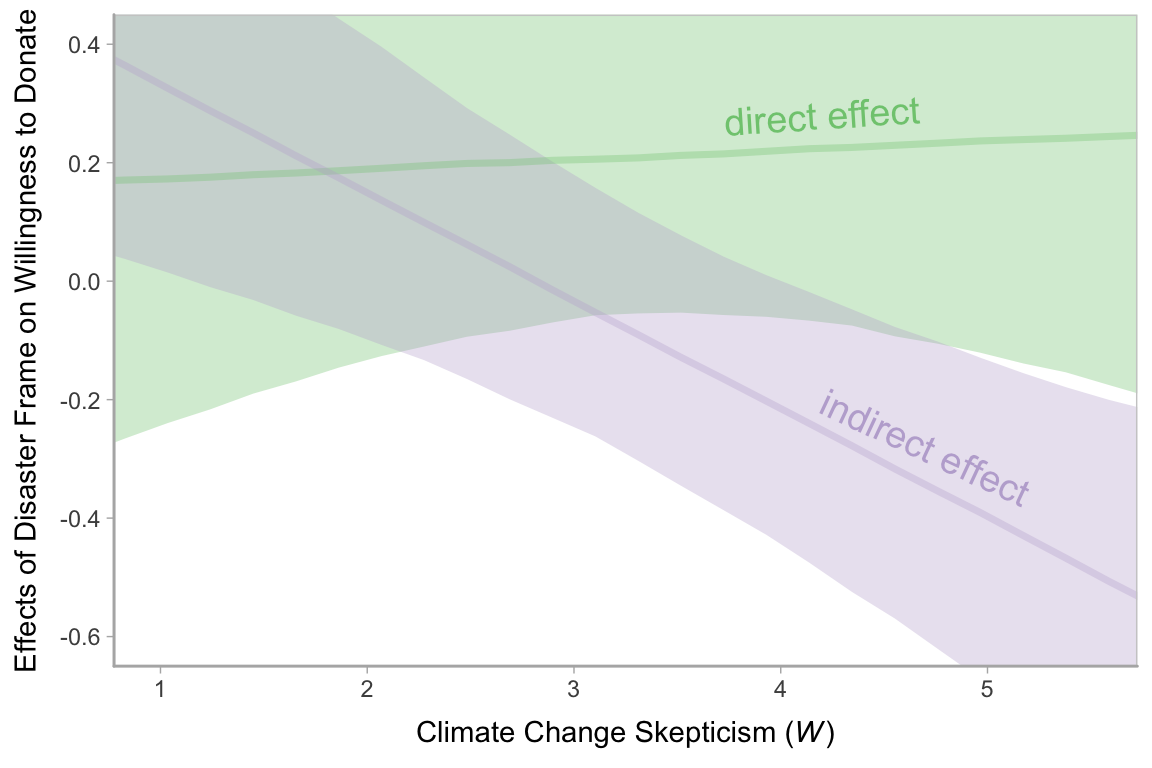## 12.3 Statistical inference

### 12.3.1 Inference about the direct effect.

We’ve already computed the 95% intervals for these. Here they are as stat_pointinterval() plots.

post %>%
select(starts_with("direct")) %>%
gather() %>%
mutate(key = str_remove(key, "direct effect when W is ") %>% as.double()) %>%

ggplot(aes(x = key, y = value, group = key)) +
stat_pointinterval(point_interval = median_qi, .prob = c(.95, .5)) +
coord_cartesian(xlim = c(1, 5.5)) +
labs(x = expression(paste("Climate Change Skepticism (", italic(W), ")")),
y = "Conditional Direct Effect of Disaster Frame on\nWillingness to Donate")### 12.3.2 Inference about the indirect effect.

#### 12.3.2.1 A statistical test of moderated mediation.

To get a sense of $$a_{3}b$$, we just:

post <-
post %>%
mutate(a3b = b_justify_frame:skeptic*b_donate_justify)

post %>%
select(a3b) %>%
summarize(median = median(a3b),
sd = sd(a3b),
ll = quantile(a3b, probs = .025),
ul = quantile(a3b, probs = .975)) %>%
mutate_if(is.double, round, digits = 3)
##   median    sd     ll     ul
## 1 -0.182 0.055 -0.295 -0.082

We might use stat_pointintervalh() to visualize $$a_{3}b$$ with a coefficient plot.

post %>%
ggplot(aes(x = a3b, y = 1)) +
stat_pointintervalh(point_interval = median_qi, .prob = c(.95, .5)) +
scale_y_discrete(NULL, breaks = NULL) +
coord_cartesian(xlim = c(-.5, 0)) +
labs(title = expression(paste("Coefficient plot for ", italic(a), italic(b), " (i.e., the index of moderated mediation)")),
x = NULL)#### 12.3.2.2 Probing moderation of mediation.

As discussed in my manuscript for Chapter 11, our Bayesian version of the JN technique should be fine because HMC does not impose the normality assumption on the parameter posteriors. In this instance, I’ll leave the JN technique plot as an exercise for the interested reader. Here we’ll just follow along with the text and pick a few points.

We computed and inspected these 95% intervals, above. Here we look at the entire densities with geom_halfeyeh().

post %>%
select(starts_with("indirect")) %>%
gather() %>%
rename(indirect effect = value) %>%
mutate(W = str_remove(key, "indirect effect when W is ") %>% as.double()) %>%

ggplot(aes(x = indirect effect, y = W, fill = W %>% as.character())) +
geom_halfeyeh(point_interval = median_qi, .prob = c(0.95, 0.5)) +
scale_fill_brewer() +
scale_y_continuous(breaks = c(1.592, 2.8, 5.2),
labels = c(1.6, 2.8, 5.2)) +
coord_cartesian(xlim = -1:1) +
theme(legend.position = "none",
panel.grid.minor.y = element_blank())### 12.3.3 Pruning the model.

Fitting the model without the interaction term is just a small change to one of our formula arguments.

model4 <-
brm(data = disaster, family = gaussian,
bf(justify ~ 1 + frame + skeptic + frame:skeptic) +
bf(donate ~ 1 + frame + justify + skeptic) +
set_rescor(FALSE),
chains = 4, cores = 4)

Here are the results.

print(model4, digits = 3)
##  Family: MV(gaussian, gaussian)
##   Links: mu = identity; sigma = identity
##          mu = identity; sigma = identity
## Formula: justify ~ 1 + frame + skeptic + frame:skeptic
##          donate ~ 1 + frame + justify + skeptic
##    Data: disaster (Number of observations: 211)
## Samples: 4 chains, each with iter = 2000; warmup = 1000; thin = 1;
##          total post-warmup samples = 4000
##
## Population-Level Effects:
##                       Estimate Est.Error l-95% CI u-95% CI Eff.Sample  Rhat
## justify_Intercept        2.454     0.149    2.161    2.745       3240 1.001
## donate_Intercept         7.258     0.234    6.813    7.726       4000 1.000
## justify_frame           -0.565     0.219   -1.003   -0.144       2774 1.002
## justify_skeptic          0.105     0.038    0.033    0.180       3178 1.001
## justify_frame:skeptic    0.202     0.056    0.094    0.311       2568 1.003
## donate_frame             0.208     0.143   -0.071    0.488       4000 0.999
## donate_justify          -0.918     0.083   -1.083   -0.759       4000 1.000
## donate_skeptic          -0.037     0.037   -0.109    0.037       4000 1.000
##
## Family Specific Parameters:
##               Estimate Est.Error l-95% CI u-95% CI Eff.Sample  Rhat
## sigma_justify    0.818     0.040    0.744    0.900       4000 1.000
## sigma_donate     0.986     0.050    0.896    1.091       4000 1.000
##
## Samples were drawn using sampling(NUTS). For each parameter, Eff.Sample
## is a crude measure of effective sample size, and Rhat is the potential
## scale reduction factor on split chains (at convergence, Rhat = 1).

Since we’re altering the model, we may as well use information criteria to compare the two versions.

loo(model3, model4)
##                   LOOIC    SE
## model3          1117.48 33.12
## model4          1115.41 33.13
## model3 - model4    2.07  0.53

The difference in LOO-CV values for the two models was modest. There’s little predictive reason to choose one over the other. You could argue that model4 is simpler than model3. Since we’ve got a complex model either way, one might also consider which one was of primary theoretical interest.